Select Page

# Enter the CosmosThis article continues from the previous one (The Antidote and the Antivenom—Decoding the Cosmos), which must be read prior, as it builds upon the material therein presented.  These two articles are a repose from the year long journey into the inner cosmos that we have been sojourning together. It is a necessary so that people can catch up and get up to speed before soaring. While we briefly touch upon numerous points previously covered in depth, these articles are not recaps; they are necessary preparation and chock full of new material and concepts. Our faithful companions along our journey, the clear mirror, aspeklaria h’meira (אספקלריאה־המאירה) and the Still Small Voice are still with us and are still teaching from places beyond our comprehension, just the wacky and otherworldly visual cues have been omitted for now.  These articles will complete Chapter 32 and I highly suggest anyone who has not been on this journey to go back and read at least Chapter 32 and The Journey contained therein from the beginning and use the search tool to investigate the prior 31 chapters of The Ultimate Reality for a clearer understanding on any particular point or concept.

Before we can move deeper, we must understand the nature of the gematria ciphers of both the cosmic kind and the Hebrew Alef-bet interface kind, and their role in decoding the inner and outer cosmos with their hyperspace nature.

There is a another gematria sequence that JD and I were working on, but it is more like an equation built into the Torah and the 42-Letter Name than a sequence that can be universally applied. Since each gematria cipher can be attached to a specific network of results we should be careful in discerning between the two types.  Moreover, no gematria cipher should be universally applied. They are all filters that reveal certain specific underlaying connections and conditions that exist but may otherwise be unseen. Today, thanks to smart phones, filters are thought of as masks that enhance one’s image or their photographs, usually of oneself. In yesteryear, photographic filters polarized or stripped away certain aspects of the light to bring forward other aspects of the subject. The same is true of the ancient and the even more ancient cosmic ciphers.

### The Genesis Code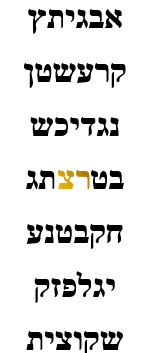As with the Pi (π), Phi (φ), Primes, and Integers or Triangular Number ciphers we are still taking 27 consecutive digits from their respective sets and summing them up cumulatively to get a new assigned value for each of the 27 positions of the Alef-bet. In the case of this new cipher though we are starting with the 2nd letter of the Torah’s first verse, Resh (ר) and ending with its final letter Tzadi sofit (ץ), the 28th. At first glance this may seem counterintuitive since in all the other cosmic ciphers we began with the first digit; nonetheless, the reason for this is that in the exact middle of the 42-Letter Name Matrix is the letter pair Resh-Tzadi (רצ) and we know that the first 42-Letters of the Torah were derived from the 42-Letter Name. These are also the final two letters (רץ) in the Torah’s first verse. Those 2 letters (רצ) with their ordinal positions in the verse have a complete value of (290 + 55) = 345, that of Moses.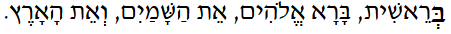To assign numbers to the letters in this first of all verses we used their katan or reduced values, giving them single digit assignments. This is the same numeric assignment that gave us 358.777 or Moshiach when multiplied by Phi (φ). As such, the final letter in the Alef-bet, Tzadi sofit (ץ) winds up having a value of 80, as in the 80 times that standard value 358 of Moshiach appears in the Torah.

When this gematria, which we will call Genesis (רץ), is applied to all 27 Alef-bet positions, then added to the Phi (ϕ) cipher, the result is 2889, as in the 2889 HC midpoint to 5778 and to King David’s life. This is cosmically fitting and satisfying as this gematria cipher is based on the midpoint of the 42-Letter Name Matrix.

It should also be noted that consequently 2 (Genesis (רץ) + Phi (φ)) ciphers equally 5778, thus this equation is associated with the other Spherical Time equations that include 5778, the value 2 and both Pi (π) and Phi (φ), though this time we have reverse engineered it to see how they are backed into the Torah’s first verse.  When its ordinal value (27) is added to complete the 27th letter Tzadi sofit (ץ) in this cipher, its letter value goes from 80 to 107, as in the 107th Triangular Number, 5778.

Taking it a step further we see that the sum of the 26 logarithms for the 26 positions of the (Genesis (רץ) + Phi (φ)) cipher starting with the Alef (א) of Bereshit is precisely 50.050500. This is extraordinary in its simplicity of outcome and in its complexity of design. It is a representation of one-half, or 50%, as in halfway to 5778 and halfway through the 42-Letter Name Matrix.Moreover, when this new cipher is applied to the 7th and finale level (שקוצית) of the 42-Letter Name Matrix its resultant value is exactly 1618, or Phi (ϕ), the same value as the 7 Triplets of the fiery serpent. As we have recently discussed the two complementary landing Triplets to the head and tail of that fiery serpent each have a value of 500, as in 50.050500. Once again, this associate with Phi (ϕ), the Torah’s first verse, and the 42-Letter Name Matrix is astounding, but its roots go even deeper.

Phi (φ) can be derived as (√5 + 1)/2 and 1/(φ) = (√51)/2, bringing together the concepts of 5, ½, and 1: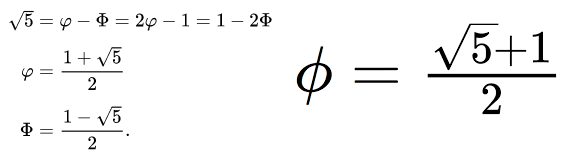Thus, we see the deeper aspects built into the design of the 42-Letter Name Matrix whose 2 landing Triplets equal 1000, or 500 each, the pole on which the fiery serpent of Phi (φ) or 1618 is split.

Now considering that we are dealing with the first 7 words and 7th Triangular Number (28) in letters of the Torah, and the 7-tier (7-dimensional) structure of the 42-Letter Name Matrix, and in particular its 7th tier, and considering that our mathematics on calculated with base 10,  it is noteworthy that 5005 is the 7th order of the summation series, rank 10th rank in Pascal’s pyramid. As previously discussed, this number in the pyramid is right after 3003, the sum of the first 8 words, or 33 Letters, the 77th Triangular Number.

Yet another connection between the networks is that the collective frequency of the 1820 YHVH (יהוה) in the Torah is (1820 x 27.5 Hz) = 50050.

### The Physics of Adam and Eve

The value 2π is integral to all our physics and to Spherical Time, so it is unbelievably significant that the complete cipher for the cumulative first 27 digits of 2Pi (π) equals 4222, the hyperspace counterspace to 5778, in other words 1054222 = 5778.

### C = 2π(5778) = 36304.24470

As previously discussed, the level and aspect of the YHVH (יהוה) that applies to the Man (Adam) are the 6 bundled dimensions of Zeir Anpin known metaphysically as (יוד־הא־ואו־הא), of numerical value 45.  In an even deeper concept, the kabbalists explain that the Names consists of a front (יהוה), kabbalistically a male aspect, which is also the original Name, and a back (וד־א־או־א), kabbalistically a female aspect, which is the spelling out of the Name, or numerically 26 and 19 respectively.

Reinforcing these conclusions is that Adam and Eve, or numerically (45 + 19) = 64, which is 26, representing the 6 dimensions of Zeir Anpin. As it happens, there are exactly 19 free parameters or measured physical/mathematical constants that are necessary for our known universe to exist. In other words, if you change even one of these 19 parameters, or measures of the fundamental particles and forces, the universe never would have sprung into existence in a form anywhere near to what we know today, no life as we know it, no planets, etc.  Of these 19 unchangeable parameters 10 consist as mass: the mass of the Up, Down, Charm. Strange, Top and Bottom Quarks; and the mass of the Electron, Muon, Tau and Higgs. The other 9 of the 19 parameters deal with energy and rate of decay: the decay rate of the Down, Strange, Charm and Bottom Quarks; the strength of the Strong Force, Weak Force, Electromagnetic Force, and the Higgs Field; and the QCD parameter.  Of the 45 total standard value in Man (אדם), 19 correspond to Eve (חוה), representing physically. These 19 correspond to the 19 parameters of physicality. The other 26 correspond to the essence unknown, the ether, the forces and pressures and fields whose interactions create that scaffolding to physicality, in the same way Adam gave way to Eve, the 19 parameters of physicality.

The ratio of the Eve to Adam proportion or Eve/Adam is 19/45, which is 42.22%, which means the Creator to Adam (26/45) proportion is 57.78%. Thus, the YHVH (יהוה)/(יוד־הא־ואו־הא) or Front/Whole is 57.78%, and the Back/Whole is 42.22%.

Moreover, as the Adam and Eve ratio breaks down to 19/45 or 42.22% and 57.78%, so does the Name YHVH (יהוה) with its ratio of (וה) and (יה) or 15 and 11, as in the 15 Triplets of the Shema and the 11 Triplets of Bereshit.  The ratio of 11/26 and 15/26 works out to .423 and .577 respectively.

### The Speed of Light

As previously explained, the two sides of Spherical Time, the equal distant radii of 2018 years of physical time and the 3760 years of spiritual time are set in a proportion that is based on the exact speed-of-light in mps, and we have recently seen how Pi (π) and Phi (φ) are entwined and integrated into the 42-Letter Name, the Torah, the Alef-bet, and naturally our physics, so it is not surprising that (1/φ – 1/π) = .2997241.. which is off from the perceived speed of light, 299,792,458 m/s by only 48 m/s.  Meanwhile, (1/φ – 1/π)/(1/42 – 1/48) = 100.707 as in the exact 107,007 letter where we find the 10 Commandments. Moreover, the 42-Letter and 48 Letters of the 12 YHVH are the two matrices at the center of the Cosmic Wheel (discussed throughout Chapter 32), just like the total of the 22 Names of the Alef-bet, 4248.

As an added synchronicity, the sum of the Pi (π) and the Prime Number ciphers for the 27 positions of the Alef-bet is 2997, as in the speed of light, 299,792,458 m/s, and as in the counterspace analogue to 3003, the sum of the 11 Triplets of Bereshit.

### More Cosmic Connections

Sometimes the ciphers are meant only to reveal specific equations, a specific narrow window into the consciousness of the cosmos. Nonetheless, the true ciphers form networks of values. Most of the values in these networks are somewhat meaningless to us even if they do equate to some valid word or phrase; after all, there are 210 standard word values in the Torah alone.  When we superimpose the various and disparate cipher nets, they form a super network of the nodes where they intersect. This, in turn, reveals what is really being conveyed.  Think of it as one super network of neural consciousness and each of the individual cipher networks that we see is nothing more than a shadow cast from a light beyond that super network at a specific angle.

What are the numbers found in common most often in most of the networks, and how do those nodes connect with one other? These interactions are the real relationships that matter.

If this all seems too abstract for you, just ask yourself if this is so different than how the disparate chains and networks of neurons in your brain work? There are 86 billion of them and for the most part, they all engage and disengage at the appropriate moment, communicating nearly instantaneously, all the while making sure the 37 trillion cells and innumerable systems in our bodies are also towing the line and doing their thing correctly. And like the cosmic networks of consciousness, our brains also operate at specific frequencies.

### The Pi (π) Cipher

In the previous article we repeated how 5 basic elements of the Torah sum to 401273, which when divided by Phi(φ) equals 248000, and that the sum of the 5 Names of the 5 Books of Moses is 2480. What we did not mention is that the 1024th Triangular Number, in other words the sum of the 1024 positions of the 1024-word values in the Torah, is 524800, or that the Pi (π) cipher gives a valuation of 2480 to the 42-Letter Name.

Moreover, two of the Triplets (צית and יתצ) in its’ 1st and 7th tiers, each attain the value of 273, the cosmic harmonic that our entire solar system is built upon, as in 401273.  And because the 7th tier has a Pi (π) cipher value of 448, as in the 448 edges of the 7-dimensional hypercube, this means that the remaining Triplet (שקו) on the 7th tier, the tail of the fiery serpent, has a value of 175, as in Abraham’s age, which is fitting considering his standard value is 248.

### The Alef-bet Hypercube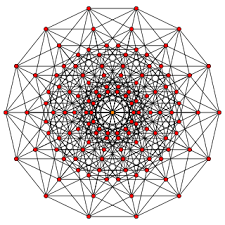Everything is symmetric in the upper hyper-dimensions. If we were to continually expand the spelling out of the 22 letters to their 22 Names and then to the Names of the 61 letters in those 22 Names, then again and again to 7 levels, the average number of letters derived from the original 22 letters on this 7th dimension is 448, perfectly matching the 448 edges of the 7-dimensional hypercube, one letter per edge.

To be clear that is 9856 letters in total, divided equally into 448 edges of 22 letters each, or alternatively, each of the 22 letters expanded into a separate 7-dimensional hypercube of 448 edges. One letter per edge, one hypercube per letter.

The 22 Letters of the Alef-bet are projected downward through 7 dimensions as a condensed version of the 448 letters of the 448 edges.  These are 4 edges/lettersfor each of the 112 Triplets, the 4 edges of the Tetrahedron, 112 Tetrahedra in total over 7-dimensional space.

Within that progression, the first letter, Alef (א) goes from 1 letter to 3 to 8 to 21 to 54 to 137 to 345, the numerical value of Moses. As the number of letters in the Alef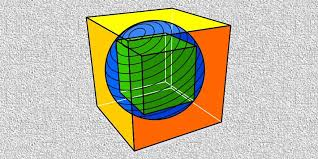(א) concentrate from the nodes connected to Moses (345) to Kabbalah (137) and the Fine Structure Constant, to the 54 cycles and portions to Ehyeh (אהיה), the Name of the 8 dimensions, to 8 to One (1), the actual number of Alefs (א) amongst those letters descends geometrically from 32 or 25 to 24 to 23 to 22 to 21 to 20 to 1 for a total of 64 or 26. The total value of those 345 letters on the 7th level is 21,888 or (22,000112). As they concentrate, they descend from (22,000112), and their cumulative values descend from 1424, the value Kadosh Kadoshim (the Holy of Holies cubic chamber at the core of the Holy Temple) and the 1.424 ratio of the cube within a cube, to 378 on the 3rd level or 3-dimensional cube to 112 to 1.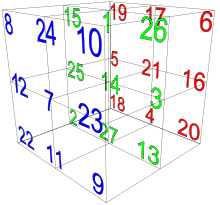As everything ties into One, we see that the cube root of 2889, the demi-radius of the Spherical Time radius (5778), representing the year 2889 CE, the exact midpoint in King David’s life, is 1.4242378, as the Alef (א) expansions from 1 to 112 to 378 to 1424, and the Holy of Holies, designed by King David, whose numerical value is 14, as in the central position (14) of the Alef-bet and Essential Cube of Creation (378), designed to equal 42 from every aspect.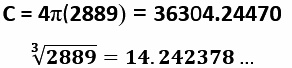The katan (reduced) value for those 345 letters is 1370, connecting to the Fine Structure Constant (137.0) and so much more.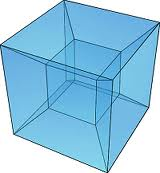Thus the 3-dimensional cube level the Alef (א) contains 12 cumulative letters, as in the 12 edges of the cube, and 8 static letters, as in the 8 vertices or corners, containing the 112-valuation, just as in the 8 corners of the Essential Cube of Creation, while the total cumulative value of the Alef (א) cube is 378, also as in the Essential Cube of Creation.

Everything comes from One.

The total cumulative value of the 1973 letters through the 5th expansion is 100,002 or (105 + 2). Rav Brandwein, of blessed memory, explained to me that the time of Moshiach Ben Joseph ended in 1973 with the ’73 War, but whether these cosmic events are connected we may never know, yet we can see the Hand of the Creator in the 105 total valuation.  That it is off by 2 and not precisely 105 appears to be a calling card of the Creator, like the trail of breadcrumbs our consciousness leaves for us across the paths of time, puzzle pieces to be fit together and guide us forward. This is no different than the letter pairings of the 231 Gates of Wisdom, whose sum of their occurrences in the Torah is 222,004, off by 4, or to the 10 Commandments being found at letter 107,007, off by 7, and that the sofit sum of the 166 letters of the 3rd expansion of the 22 letters is (22,0006). The Creator has shown that He can make any value in the Torah or the universe from the distance between the planets, the temperature on the surface of the Sun, to the measure of the Plank Constant and the digits in Pi (π) be as exact and precise as He chooses, so when He chooses not to, we should pay close attention.  Perhaps it ties into the sum of the collective ordinal positional values through the 5th word, the 21st letter, in the Torah being 231.

While the 166 letters of the 3rd expansion of the 22 letters is (22,0006), their initials total 4248, and their 44 middle letters total 666.

The 5th expansion, whose cumulative sum totaled (105 + 2), has a sofit value of 126,778 for its 1267 letters with an ordinal value of 13,333 or (40,000/3) for a complete value of 140,111, which is 132 less than the total value of all the letters Hei(ה) in the Torah, 140,280, the 5th letter in the Alef-bet.  Its 353 middle letters total 5780. The Creator made sure we would understand that none of this is not coincidental, as He made the EB-GD (shift -1 letter higher) gematria value of the same 5th expansion letters equal to 23200, as in the 4 aspects (232) of the YHVH (יהוה).

### The Primes and Integer Digit CipherBecause they interface through the 27 positions of the Essential Cube of Creation the cosmic ciphers are all based on the full 27 letter positions of the Alef-bet interface, which are based on their 22 Names and include their 5 final (sofit) letter variations. Another extremely revealing cipher is the combination of the cumulative first 27 Prime Numbers along with the cumulative first 27 digits in the set of all positive integers.

The sum of this Prime-Integer Digit cipher when combined with the Pi (π) cipher gives a total of 3141, as in Pi (3.141…) for those 27 digits, but the combination of the Primes and Integers digits on its own is just as revealing.

For example, utilizing this cosmic cipher the sum of the 27 letters gives us a total value of 2533, as in the sum of the integers from 1 – 22, the 22nd Triangular Number, 253.

There is one extremely significant node that glows brighter and brighter with each overlapping cipher network or superimposed web. This is the Triplet Yabok (יבק), that normally has the numerical value of 112 but with the Prime-Integer Digit cipher becomes 222, as in (60005778) = 222. This equation is cosmically significant as the 6000 years represents Zeir Anpin and 222 are the number of years the Event Horizon is designed to be off the galactic 6,000-year half cycle. Moreover, as Rav Ashlag explained, 222 is the discarded remnant of sequentially applying the .666 ratio to the 6000 years in order to separate the spiritual from the physical:

6000.00 =        4000.00  +  2000.00

2000.00 =       1333.33   +    666.67

666.67 =         444.44   +   222.22

5777.78    +   222.22 =      6000.00

There is much more to it than that and we will discuss Yabok (יבק) and its prominence in more detail shortly. Of consequence, though, is that 222 as a percentage of 5778, or 222/5778 = 3.84216%, as in the standard numerical value of the first 42 Letters in the Torah, associated with the 42 Letter Name, 3842.

What is especially revealing about the Prime-Integer Digit cipher is that when applied to the 42-Letter Name its total value becomes exactly 3842, as in the first 42 Letters in the Torah.

Moreover, its first Triplet (אבג) attains a value of 20, as in the letter Caf (כ) for the Sun, and its 2nd Triplet (יתצ) attains one of 420 as in the Sun (כ) and Moon (ת) together, and its 7th tier (שקוצית) has the value 702, that of Shabbat, as in the Great Shabbat of the 7th millennium.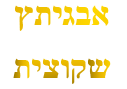When we speak about the networks converging on specific nodes, it is because with each added and descending dimension the networks expand outward, and their superimposition implies that we are looking into a higher and thus narrower dimensionality.  At this higher level each node or major juncture is highly focused.  For example, the sum of the 27 positions of the Genesis (רץ) cipher is 955 and the sum of the 5th and 6th tiers of the 42-Letter Name is 955 when utilizing the Prime-Integer Digit cipher. Mathematically and physically, there is no correlation, yet we know the Genesis (רץ) cipher is derived from 27 letters of the Torah’s first verse, and that the Prime-Integer Digit cipher has shown us that the 42-Letter Name is integrally connected to the first 42 letters of the Torah that include that verse. Still, there is no causality there.  Nor is there a correlation with the 955 verses in the Torah’s 5th Book, Devarim.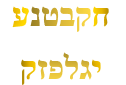It can only be that the 42-Letter Name exists on a higher dimensionality than the Torah and the Alef-bet interface, and that it is actively influencing both.

This is a key point, concept, and principle. Do not miss it. The 42-Letter Name is actively influencing the Alef-bet and the Torah. We speak about a living Torah, not a static one. The words and letters do not change; they still and always have formed geometric multi-dimensional networks of 210, 58, 223, E8 lie groups etc., but the zones and nodes that are illuminated are guided from above shift with the pathways of time. Our interaction with them influences their positioning, as does the mechanism of the cosmic wheels, and as does our generation and its needs.  The 42-Letter Name is the remote control, your smart phone to the internet of the upper-dimensions, the universal consciousness.

As a reminder if we add the Phi(φ) cipher to the 955 of the Genesis (רץ) cipher, we 2889, the midpoint radius of Spherical Time. Likewise, if we add it to the 5th and 6th tiers of the 42-Letter Name, we also get 2889 HC. We have seen that in yet another related network that the 2 (Genesis (רץ) + Phi (φ)) cipher for the 7th tier is equal to 1618 or Phi(φ). Therefore, the superimposition of networks for the 3 final tiers of the 42-Letter Name relates Phi (φ) with the 2889-year radius of Spherical Time. This makes perfect sense since the standard value of these 3 levels (239 + 230 + 906) = 1375, the Phi(φ) angle (137.5o) at the core of Spherical Time. It should be pointed out that these are the 3 levels below/beyond the center (רץ).We thus see how assorted numbers that may mean nothing to us at all, like 955, 230, or 239 when traced upward through their networked connections lead to powerful cosmic juncture points with profound implications for our consciousness and existence. It is already readily apparent that the 42-Letter Name hyper-network has a most prominent role in the design, architecture, and consciousness of our universe.

### Two AxesAs we know, the 112 Triplets are divided along the 2 axes of the primordial Alef (א). Along one axis are the 11 Triplets of Bereshit and the 15 Triplets of the Shema, which collectively add up to 26, as in the YHVH (יהוה). Their 26 initials total 1273, as in the 401273 total core elements of the Torah, and as in 1.273, the cosmic harmonic, the ratio of the square that encapsules the inscribed circle, and consequently 4/Pi(π), the skeletal framework of our solar system.

The other axis contains the 14 Triplets of the 42-Letter Name and the 72 Triplets of the 72 Names, which together add up to 86, as in Elohim (אלהים).  To the ancient kabbalists this meshing of the two Names, the YHVH (יהוה) and Elohim (אלהים) has always been a powerful and important unification, known as Yabok (יבק) of numerical value 112. The 72 initials of the 72 Triplets, which are the 72 letters of the 72-letter verse Exodus 14:19, equal 2672, nicely reflecting three of the 4 sets of Triplets and two of the Holy Names. When the two sets of initials of this axis are summed up the 86 of them equal 3732 or (3842110), with 110 Hz being the first convergence point of the Primal frequencies (1.1 Hz and 27.5 Hz).

Altogether, the 112 initials of the 112 Triplets total 3732 + 1273 or 5005, and as we just relearned, 5005 is the 7th order of the summation series, rank 10th rank, 70 levels deep, and the collective frequency of the 1820 YHVH (יהוה) of the Torah, or (1820 x 27.5 Hz) = 50050.

### The Cube of 27.5 Hz

We know the Primal Frequency (27.5 Hz) as the sacred ancient and future cubit of 27.5.” But that is in 2 dimensions. What is its configuration in 3-dimensions? Afterall, the encasing pyramid with its central Tower of Truth is a 3-dimensional structure built using the cubit as its basic dimension and the cube as its basic design feature or building block. So were the two Holy Temples, the Mishkan of 42 Elements, and the Future Holy Temple, as explained by the Ramchal. It seems like a natural transition to go from a cubit to a cube. All we have to do is calculate 27.53 and we have the volume of the cubic cubit. One cubic cubit (1 cu3) equals 27.53.

But what of the cube whose volume equals 27.5?

Each side length of that fundamental cube would be 327.5, and the area of each face would then be 9.1107709.

Let us say that that side length was instead the area of a circle, 327.5.  If 327.5 is the area of a circle, then the square the inscribes that circle has an area of 1.273 or 4/π times 327.5.

What we see when the cosmic harmonic is multiplied by the cube root of the Primal Frequency, 1273 327.5 = 3842, the first 42 Letters of the Torah. More specifically, it is 3842.43003, adding the linked networked concepts of 424, Moshiach Ben David, and the 3003 total of the 11 Triplets of Creation, the first 33 of the first 42 letters that total 3842.The value 378 is the gematria of the powerful phrase tied to Moshiach, “He and His Name are One (Echad Usho Echad)”, and there are 181 digits in 111! (factorial) with 111 being the numerical value of Alef (אלפ), One (1). And while the sum of the digits through 181 (the 42nd Prime Number) is 378, the 27th Triangular Number and (9 x 42), we know that this structure is built into the Essential Cube of Creation.

When that special fusion of the 27 positions that represents the Essential Cube of Creation, the Cube of Oneness, is divided by the Primal Frequency (27.5 Hz) what is revealed is that 378/27.5 = 13.75 or the Phi(φ) angle (137.5o) at the center of Spherical Time. It is also equivalent to 27.5/2 or half the Primal Frequency, meaning the Primal Frequency (27.5 Hz) is twice the Phi(φ) angle (137.5o), and that 27.5 x 27.5/2 = 378 = 27.52/2, or half the base (27.52) of the encasing pyramid. “Time, times, and half a time.”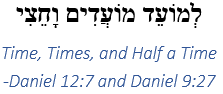As previously explained, the mysterious end-times verse in Daniel 12:7, contains the phrase, “(לְמוֹעֵד מוֹעֲדִים וָחֵצִי) time, times, and half a time,” as in 1273, and it is repeated in Daniel 9:27, as in the 9 planes and 27 positions of the Essential Cube of Creation. And while the value of this verse, 434, equals that of the Name of the letter Dalet (דלת) or 4, its square root is 20.83, as in the 7 Triplets of the 42-Letter Name Matrix that add up to 2083, the 314th Prime Number, representing Pi (π), the other primordial constant at the center of Spherical Time. Thus, the equation 4/π = 1.273 is built in.

As Rav Brandwein explained, the final letters in a phrase point to the future and these 3 letters add up to 54. And at the end of days, the circle of 54 cycles/portions of the Torah and the 54 cycles of 107 years, divided by Moshiach Ben David show us that 54/424 = .127358, as in the cosmic harmonic 1273 and Mashiach (358).

### Yabok (יבק)

As just noted, the unification of the 2 Essential Names YHVH (יהוה) and Elohim (אלהים) that equal 112 has long been known to the kabbalists. As have the 3 Essential Names Ehyeh (אהיה), the YHVH (יהוה), and Adonai (אדני) that also equal 112. This metaphysical junction of the Names in the hyper networks of the upper cosmos is obviously very powerful. It gave rise to the 112 Triplets.

The kabbalists had a name for this confluence, the river Yabok (יבק), where Jacob (יקעב) defeated the dark angel using the 112 Triplets and the Names to which they attach.

Using the ultra-revelatory Prime-Integer Digit cipher, yet another multifaceted node glows brighter and we see that the new assigned values for the 3 Names in the unification of Ehyeh (אהיה), the YHVH (יהוה), and Adonai (אדני) that totaled to 112, now equal respectively (130, 161, and 190 for a total of 481, the height of the encasing pyramid and the Tower of Truth. Individually, the Name Ehyeh (אהיה) now connects to the Path of One Network and to Mt Sinai through its value of 130, and the YHVH (יהוה) now has a direct interaction with the highest aspect of the Name Ehyeh (אהיה) through its expanded name (אלף־הי־יוד־הי) of numerical value 161.

As an intersection of cosmic networks, the Triplet Yabok (יבק) is far more prolific than most. In terms of its value using the Genesis (רץ) cipher, it is 91, tying it squaring in the middle of the Path of One in the center of the Network of One, and fittingly to the unification of the YHVH (יהוה) and Adonai (אדני) or (26 + 65) = 91. The cipher of the Integer Digits likewise ties Yabok (יבק) to the Network of One as its value is 132. Its’ Triangular Number cipher gives it a value of 358, that of Moshiach in the standard interface. And while the (Genesis (רץ) + Phi (φ)) cipher gives it a value of 253, the 22nd Triangular Number, the 2 (Genesis (רץ) + Phi (φ)) cipher naturally gives it a value of 506, matching the value of the first tier of the 42-Letter Name, unconditional love, and the complete value of Moshiach ben David.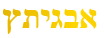These are the same two ciphers that gave us 2889 and 5778 respectively for the 27 positions of the Alef-bet.  Just to be clear, the value and concept of 27 existed long before the 10 letters of the Alef-bet expanded to occupy those 27 positions of the Essential Cube of Creation.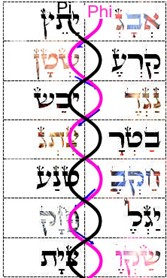The complete 2πφ cipher that also gives us 5778 for the Alef-bet when applied to Yabok (יבק) gives us 465, as in the difference between the Phi(φ) and Pi (π) serpents (20831618) = 465, entwined in the 42-Letter Name, while the complete πφ cipher value is 360, the circle.

Another synergistic cosmic intersection happens when applying the squares of the ordinal values to Yabok (יבק); it also gives us 465. This is one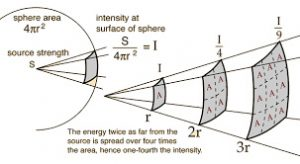of the very revealing set of the Inverse Square Law gematriot. Its basis is that a specified physical quantity or energy is inversely proportional to the square of the distance from its point-source as it radiates and dilutes into three-dimensional space.

The importance of the concept of Triangular Numbers in the Torah and Cosmos cannot be overstated, so when the Triangular Number cipher for Yabok (יבק) aligns with the value 248 the message could not be stronger.

There is a another ancient rarely used cipher, Mispar Ne’elam, which utilizes the back of the Names of the letters instead of their initials. We utilized it in separating Eve from the expanded Name of the YHVH (יהוה) associated with Adam (Man). Yet in the case of Yabok (יבק) it too gives us a value of 506, which is also the value for unconditional love. What is even more remarkable is that the milui, or fully spelled-out, Names of the letters in Yabok (יבק) is 618 or 1/Phi(φ).

There are numerous other alignments if we get into the weeds of the networks, but one important one is the complete standard gematria value of 143, as in the aspect of the Name Ehyeh (אהיה) though its expanded name (אלף־הא־יוד־הא).

### 11 Sefirot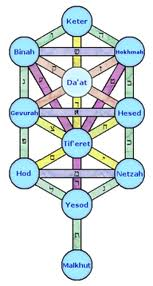One important node that appears at the prominent intersection of the concepts of Unconditional Love, Moshiach Ben David, Yabok (יבק), and the 42-Letter Name is 506. As we know, 506 is also the sum of the first 11 integers squared (12 + 22 + 33112), and of course (5 + 0 + 6) = 11, which also means that its P/S cipher ratio is 30/11, which equals 2.727272 reflecting the 27 positions and the 273 Cosmic Harmonic.Those 27 positions of the Essential Cube of Creation and ergo the Alef-bet are reflected in the 27 Chapters in the Torah’s 4th Book, Bamidbar (Numbers) of numerical value 248.  Nonetheless, because the difference between the 58 words, letters, and verses in the Torah and the 401273 or 248,000φ words, letters, verses, rows, and columns in the Torah is exactly 223 that difference is also equivalent to (113 x 8), linking back to the 248 dimensions of the E8 Lattice structure and the 8 corners of the Essential Cube of Creation that equal 112. Remember, all Torah light is spread by squaring and the square root of the powerful interface word “The Torah (התורה)” is 24.8.

We first encountered the concept of 11, as in the 11 sefirot of the Tree-of-Life, in the first pages of this ongoing book that I began with my daughter several years ago.

Using their ordinal values, we can split the 22 letters of the Alef-bet right down the middle into odds and evens, just like the Covenant of Halves, and just like the refrain in Abraham’s Sefer Yetzirah, “…with a single covenant right down the middle…”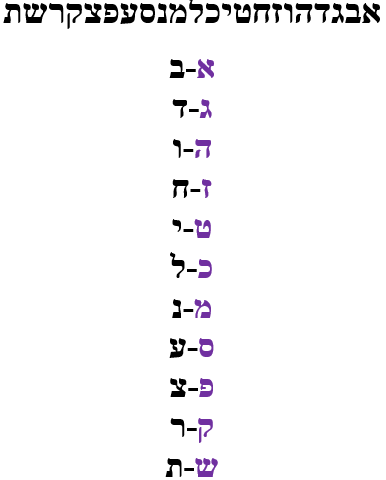There are about 5700 ways shy of 4.2 million methods for splitting the Alef-bet in 2 parts, and over 700,000 ways to split the 22 letters of the Alef-bet into two sets of 11. Of those, there are exactly 248 ways to split the letters in such a way that when their standard exponentially derived values are applied, they yield the same 42 and 72 proportions, but none of them are as elegant, simple, and clean as the 0dd-Even split and none of the others are sequential.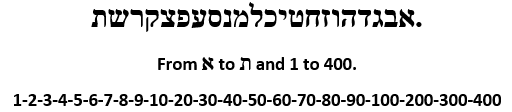When we assign these fixed number values to the split odd/even alef-bet we get: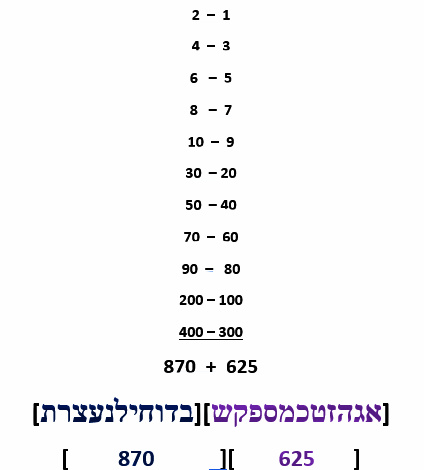The two sets of 11 letters each, which form the two column above, add up to 625 and 870 respectively for Alef-Shin (שא) and for Bet – Tav (תב) for a total value for the alef-bet of 1495. The value 625 we recognize immediately as H’Keter, the highest of the 11 sefirot and as explained what seems like ages ago, 625/1495 = 42%, reflecting the 42-Letter Names that makeup the 14 Triplets and that are included in the 15 Triplets of the Shema.  Meanwhile, the Odd set of 11 letters over the Even set of 11 letters equals 625/870 = 72%, reflecting the 72 Names/Triplets.

By now, the fact that there are exactly 248 ways to the split the Alef-bet into 2 sets of 11 letters with the proportions 42% and to 72% must seem astounding to us, especially as it extends the reach of the pure cosmic design directly into the interface letters themselves, making them reflect what is above and what is projected below.  Nonetheless, of those 248—only one of which is symmetric—there are 120 of them whose katan (reduced) values also yield the numerical value for Israel, 541.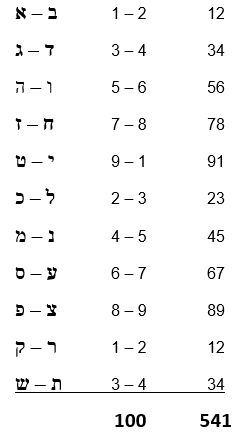The 11 pairs (12+34+56+78+91+23+45+67+89+12+34) = 541, the gematria of “Israel.” And if we add up the 22 small gematria (1 +2 + 3 + 4…3 + 4) or digit values of the 22 individual letters it totals exactly 100, as in the 100th Prime Number, 541, which is found exactly 625 times in the Torah, with 625 being the square root of the 58 words, letters, and verses in the Torah.  A design only creatable by the Creator. Cosmically, this is only possible if the same entity Created the Hebrew Alef-bet, the Torah, the base 10 numbering system, and the set of Prime Numbers, just for starters.  This is why the Torah begins with “In the Beginning G-d Created…” and why so much is packed into these 7 words.

As previously mentioned, we find that same pattern repeated in the sum of the 11 initials (דצכעדשבאחמב) of the 10 plagues, which also sum to 541, Israel, and whose ordinal values also totaling 100, and whose final 2 initials (מב) of the final plague equal 42.

Moreover, while 625 is the square root of the core Torah elements, being that it is 252 makes 25 the 4th root of the Torah’s total number of words, letters and verses. The reason this is significant is that the average of the first 25 prime numbers is exactly 42.4, yet another cosmic network alignment to the concept of Moshiach Ben David, and to the sum of all 22 Hebrew letters whose Names total 4248 and whose 22 square roots of those Names total 424.242. It also harks back to the 25 letters of the Shemas first verse, Devarim 6:4.

And these all tie back to the 11th dimension in the Tree-of-Life, Da’at (Knowledge) and the 3 initials of the central core (Keter-Da’at-Tiferet) which total 424, as in the 4 nucleotides (אכגת) of our DNA.

While that may not blow your mind, how about the average weight of those 4 nucleotides being equal to 499.5 g/mol, as in the value of the 27 letters of the Alef-bet, 4995.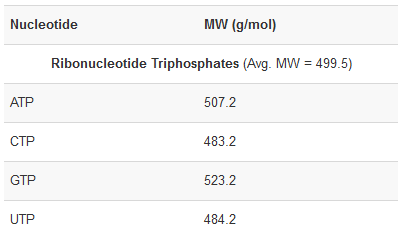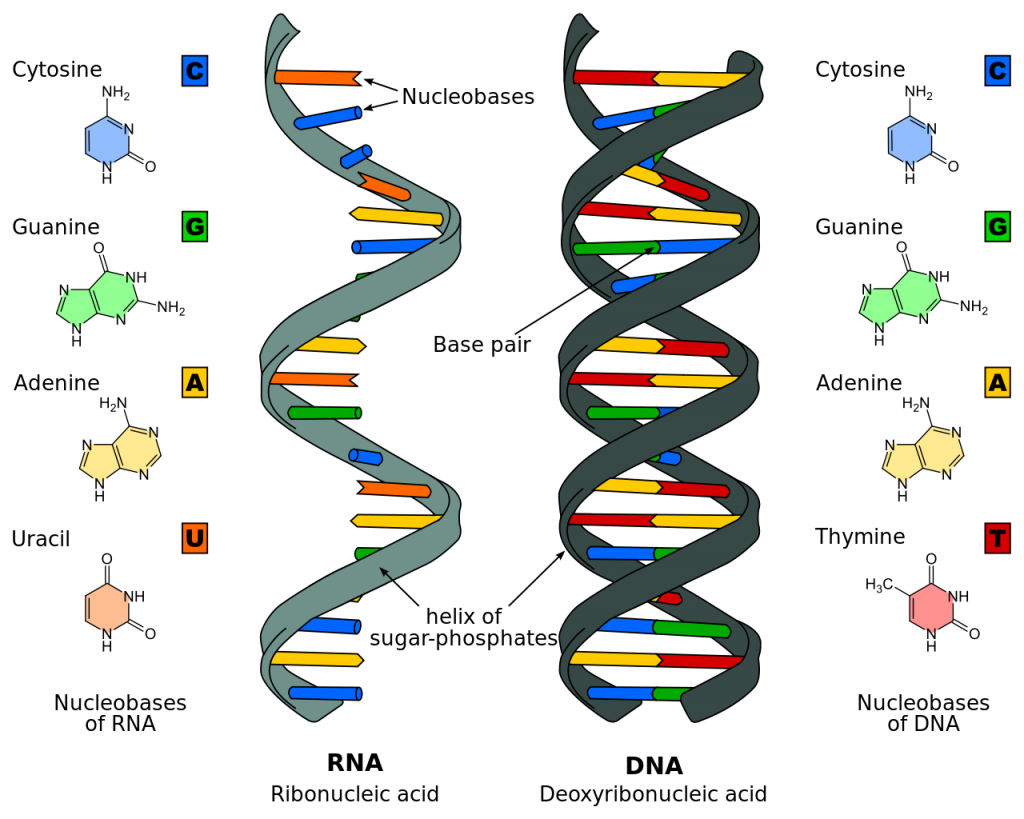And the total for the 4 nucleotides, Adenine (135.13), Guanine (151.13), Thymine (126.11), and Cytosine (111.1) divided by their initials (424) is 1.23456789, not to mention the obvious individual connections to the Networks of One (1) as in Echad (13) and Alef (111); and to Ehyeh (אלף־הה־יוד־הה) of value 151; and to the Essential Cube whose faces and planes each total 126.  Man thinks he named these building blocks and created the weight system, but the numbers were there long before Man; Man only followed the Divine forces and paths of the cosmos that led him to them.

The sum of the 27 letters in the Alef-Bet, 4995, or alternatively of the 4 nucleotides (499.5) divided by the Prime Frequency (27.5 Hz) is 181.63636, as in the 42nd Prime Number (181) and the sum (181) of the first 42 digits in the set of Prime Numbers, while the sum of the digits through 181 (the 42nd Prime Number) is 378, the 27th Triangular Number and (9 x 42). These cosmically align with the Network of One through the 181 digits in 111! (factorial) with 111 being the numerical value of Alef (אלפ), One (1).

Meanwhile, the Pi, Primes Numbers, and Ordinal value cipher of the 3 expanded iterations of the Higher Name Ehyeh (אהיה) equals 2738, as in the molecule weight of the 20 Amino Acids in our DNA, 2738.01 g/mol, which is also the Torah’s first verse 2701 and the 42-Letter Name 37.01 together, 2738.01. Coincidence or not, Tyrosine, has a molecular weight of 181.18… with tis connections to 42 just enumerated.

## Amino acids: formula and molecular weight

 Amino acid Chemical formula Molecular weight, g/mol Isoleucine C6H13NO2 131.1736 Leucine C6H13NO2 131.1736 Lysine C6H14N2O2 146.1882 Methionine C5H11NO2S 149.2124 Phenylalanine C9H11NO2 165.1900 Threonine C4H9NO3 119.1197 Tryptophan C11H12N2O2 204.2262 Valine C5H11NO2 117.1469 Arginine C6H14N4O2 174.2017 Histidine C6H9N3O2 155.1552 Alanine C3H7NO2 89.0935 Asparagine C4H8N2O3 132.1184 Aspartate C4H7NO4 133.1032 Cysteine C3H7NO2S 121.1590 Glutamate C5H9NO4 147.1299 Glutamine C5H10N2O3 146.1451 Glycine C2H5NO2 75.0669 Proline C5H9NO2 115.1310 Serine C3H7NO3 105.0930 Tyrosine C9H11NO3 181.1894

This is the same 3 iterations that when combined with the 3 iterations of the 42-Letters of the YHVH (יהוה) equals 3142. What is especially odd, or cosmically satisfying, is that the value of the Phi(φ) cipher for these 3 iterations is 1571 or Pi (3142)/2, linking the 2 primordial fields with the Name of Consciousness.  Astonishing, but no longer shocking, especially as its second iteration has a Phi(φ) cipher valuation of 363, that of H’Moshiach, which is tied into the Spherical Time formula.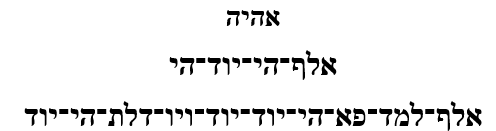But back to the superimposed nodule of 506. It is a mathematical property of summing up the consecutive odd integers that they form natural squares. For example, (1 + 3) = 4 or 22, and (1 + 3 + 5) = 9 or 32 and so forth, thus the total for the 11 odd ordinal numbers is 112.  We can thus see that 506, the value of the first tier of the 42-Letter Name Matrix, is the sum of the 11 odd ordinal cumulative sums, in other words the 11 Triangular Numbers of the set of odd integers, whose standard letter values total 625.

Meanwhile, the sum of the squares of those 11 Odd letter values is 112,165, and 216,620 for the 11 even ones, with all their obvious networked connections and intersections with the 112 Triplets, Keter (620), the 216 letters, etc.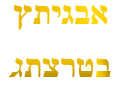Moreover, we note that the 42-Letter Name was further designed in such a way that its 1st and 4th tiers work together as (506 + 704) to equal 1210 or (112 x 10), and to intersect with the 1210 verses in the Book of Shemot (Exodus). The sum of the 40 chapters, 164 paragraphs and 1210 verses in that Book equals 1414, as in the sum of the names and ages of the 4 Patriarchs with the kolel (248 + 208 + 182 + 156) + (175 + 180 + 147 + 110) + 8 = 1414, the square root of 2: 1.41421

### The Extraordinary Essential Cube of CreationThe other 4 Essential Elements of Creation are arrays that are derived from multi-dimensional matrixes. The Essential Cube of Creation is already a 3-dimensional matrix that adds up to 42 in every direction. We have already seen throughout chapter 32 many wonderous alignments including the formation of revealing Magen Davids on each face and plane and axes that align with historic dates, including 9-11.

Of the hundred or so pages of already written about the Essential Cube of Creation, there is one correlation to the Torah we have not mentioned yet. The central position of the Cube corresponds to the letter Nun (נ) of numerical value 50 as we well know, but what we did not realize before is that there is exactly 11250 letters Nun (נ) in the Torah, as in the 112 Triplets, and the 8 corners of the Cube that surround the central Nun (נ) for a total value of exactly 7502.

As a matrix, each of the 27 or 33 positions is a coordinate in space (Column, Row, Depth), starting with (0,0,0) for the first position, the 9 of the southern face. The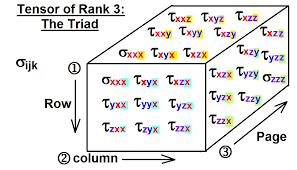number 11 beside it is the second position (1,0,0), and the 22 beside that is (0,0,2). This is a Cube interface with the Hebrew letters, so everything is ordered from right to left. The 3 planes delineated below are sliced vertically from front to back: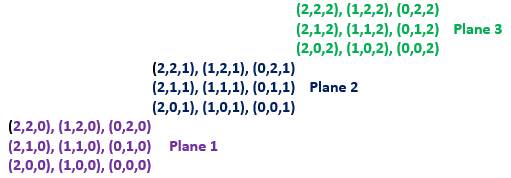Every cube has 26 elements (12 edges, 8 vertices, and 6 sides). This, being a centered cube, it has 26 rays or spokes radiating from its central position (14), thenumerical value of David (דוד), as we also find in the exact center of Spherical Time. Every letter is exactly one position (spoke) away from the center one and with simple rotation about it axes every letter can swiftly permute into any other, and every root and triplet can be created with the simple shifting of triangles, triangulated relationships.

By re-ordering the 27 positions according to the order of their coordinates and then reordering the standard values of the 27 letters of the Alef-bet to match, we can reassign their values into a new cipher (the Essential Cube cipher), whose total will still be 4995, but whose value for each word will now be completely different. The same can be said and done with their ordinal and complete values.

When the letter counts for each of the 27 letters within the Torah is redistributed according to its coordinates of the Essential Cube and then multiplied by their new ordinal values, the total ordinal values for the Torah become 3935972, as in the numerical value of the 72 Triplets with the kolel (3 x 72) for the 216 letters in the 72 Triplets, 9359. Moreover, the 8 vertices of the Cube, the 8 corners, have a complete standard value of 935.

What are the letters of the Numbers telling us? Is this even possible if both the 72 Triplets and the Essential Cube of Creation did not predate the Torah? If that is so, then the splitting the Endless Sea with its 3 consecutive verses of 72 letters that comprise the 72 Triplets must have existed (happened) prior to Creation and prior to the Torah as well.  This means that the slavery, exile, and exodus were all contemplated before even the Creation of Man and physicality.

The cubed gematria value of the 9 letters in the unification of the 2 Essential Names YHVH (יהוה) and Elohim (אלהים) that equal 112 becomes 93592. This is a unification found in the Torah, as in the expression “And the Lord G-d said (ויאמר־יהוה־אלהים)” It is first used in Genesis 2:4, the 35th verse of the Torah, immediately after the 7th Day was completed and blessed and the small letter Hei (הִ) in “were created, B’Hibaram (בְּהִבָּרְאָם)”  or Abraham (אברהם) was established, completing the large Bet (בְּ) of Bereshit -small Hei (הִ) combination (בְּהִ) of numerical value 7 that bookends the 7 Days, 7 sefirot/dimensions of Zeir Anpin and the projection toward physicality.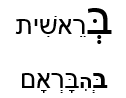Using the Essential Cube cipher, Yabok (יבק) attains the value of 65, that of Adonai (אדני), the third of the 3 components of the unification whose deeper connections were revealed. And while the ordinal value of the first Triplet in the 42-Letter Name becomes 42, and the total value of that Name becomes 6913, or Bereshit (913) plus 6000, the ordinal value of the Torah’s entire first verse becomes 345, the value for Moses, and the value for the 11 Odd letters goes from 625 to 1533, the number of verses in Bereshit.

One significant equation derived from the cipher of the cumulative positions of the Essential Cube is that the highest Name Ehyeh (אלף־הי־יוד־הי) attains a value and frequency of (42.4 x 27.5 Hz), that of Moshiach Ben David, and because of cosmic network linking and superimposition, we now know it to be that of the potential built into our DNA as well.

### 10 Utterances of Creation

Like the 10 letters (1,2,3,4,5,6,7,8,9,0) of the set of all Number, and the 10 original component letters of the Hebrew Alef-bet (דוזיכנסרךן), there were 10 Utterances from the Creator that led to the projection we call physicality. The Hebrew word analogue to these are found in the first chapter of the Torah.

As a reminder, we see that the word V’Yomer (ויאמר) is used as part of the expression “G-d said (ויאמר־אלהים)” 23 times, including the 10 times of the 10 Utterances of Creation in Genesis Chapter 1, leaving 13 for the rest of the Torah. The sofit value of the 10 letters in Vayomer Elohim (ויאמר־אלהים) is 903, the 42nd Triangular Number.

The final time this phrase appears is in Numbers 22:12 when G-d said to Bilaam do not curse the Israelites for they are a blessed nation. This is in the middle of the saga of the 3 incidents including the fiery serpent as recently discussed. This was while Bilaam tried to use the 42-Letter Name against the Israelites to no avail.

The word Vayomer (ויאמר) alone is used exactly 720 times in the Torah, which include the expression Vayomer YHVH (ויאמר־יהוה) that is found exactly 107 times, as in the 107th Triangular Number, 5778, the Spherical Time radius. Everything in the Torah and in the cosmic universe that it reflects is by design, and thus so is the equation (903 + 107) = 1010 = (10 x 101) with 903 being the 42nd Triangular Number, the 107th Triangular Number being 5778, and 101 being the 26th Prime Number.

These are the first nine of the 10 verses in chapter One, where we find Vayomer YHVH (ויאמר־יהוה): 1:3, corresponding to the 1st day of Creation; 1:6 corresponding to the 2nd day; 1:9 and 1:11 corresponding to the 3rd day; 1:14 corresponding to the 4th day; 1:20 corresponding to the 5th day; and 1:24, 1:26, and 1:29 corresponding to the 6th day.

The sum of (1,2,3,3,4,5,6,6,6) days is 36; of the verses is 142; and chapters is 9; totaling 187, as in the 187 total chapters in the entire Torah.  Meanwhile, the sum of the 9 chapters and 142 verses is 151, the value of the spelled-out higher Name Ehyeh (אלף־הה־יוד־הה) and of the word, mikve.

Each one of the Utterances begins with “Vayomer Elohim,” connected to the number 42 as we just explained, followed by a command to the elements or to the forces within hyperspace. Three of those commands were passive, as in “there shall be,” followed by a noun. If we sum those 9 commands they sum to (232 + 405 + 122 + 705 + 666 + 606 + 497 + 425 + 60) = 3718, as in the equation (3718 + 42) = 3760 (0 CE), the pivot point of the Divine Calendar, and Spiritual Time component.

The theme of 42 in Creation keeps getting stronger, and yet the sum of the final letters in those 9Vayomer Elohim” is 7200, as in the 720.0 sum of the square roots of the 72 Triplets, which is interesting since the 8th Utterance, 425 is the same as the 8th Triplet (כהת) of the 9th Candle of the 72 Triplets, which know predates that Torah.

On the other hand, when we add these 9 Utterances without the 3 passive “There shall be (יהי)” and just leave the nouns–Light, firmament, and lights—the total numerical value including the 187-placement value delineated above is 3830, as in the year the final exile began with the destruction of the Second Holy Temple, in 70 CE.

Of these 9 Utterances, the first one is logically the 232-value of “There shall be Light,” as in the sum of the 4 Aspects of the YHVH (יהוה), and the 5th one, “There shall be [heavenly] lights,” is 666, but the 6th one, or 606, is even more revealingThe sum of all 9 of those Vayomer Elohim or 9 x 903 is 8127 and we find the numerical string …8127… at the 606th digit in Pi. Moreover, the sum of all the digits in Pi counting backward from 1000 through the 8127 string is 1775, as in the sum of the 27 letters of the Hebrew Alef-bet, 1775, whose square root is 42.130… or 42 at Sinai (130), and a prominent nodal point between the Path and Network of One, the Network and singularity of 42, and as revealed by the field of Pi (π), the Alef-bet and the 10 Utterances of Creation.

What is more is that 8127 is also the determining factor in the equation 9/(42-Letter Name katan or .123049) = (70 + π), in that when we flip the equation we get 73.14159/9 = 8.127.

Think about the cosmic planning involved in all this. What is more is that these equations were translated into the Hebrew interface and set into specific places within the Torah Matrix: so that 9 of the 42nd Triangular Numbers (903) would equal a specific numerical string (8127) in the first 1000 digits of Pi (π), so that its remainder digits would equal the sum of the 27 Letters of the Alef-bet; and that (70 + π)/9 would equal 9 of those 42nd Triangular Numbers, and thus that 1/((70 + π)/9) would equal the katan string of the 42-Letter Name of G-d.

Not to mention that this very specific place within Pi (π), is 27 digits before digit #633, where we find that first of 3 strings …5778…. And that while the sum of the cumulative total for the first 27 digits of Pi (π) is 1733, as in the small gematria of the 42-Letter Name (173), this is exactly 42 less than 1775, the sum of the 27 letters of the Hebrew Alef-bet.

So, what about the 10th Utterance? When we sum the (1st, 2nd, 3rd, 3rd, 4th, 5th, 6th, 6th, 6th, and 6th) days we get 42 once again. And when the verse at 1:28 is added we get 42 total days, 180 chapters-verses for a new total for the 6 Days of Creation of 222, or (6000222) = 5778. It must be noted that 222 is 1/27th 6000, or 6000/27, as in the 27 positions of the Alef-bet and Essential Cube of Creation.

The 21 initials of the 9 Vayomer Elohim and 10th Vayomer Lahem Elohim is (63+37) = 100, as in the ordinal value of the first 10 letters of the Torah, and as in the 100th Prime Number, 541. Israel.

The value of the Name Ehyeh (אהיה) is 21, and 21 of those 23 times are found in Genesis, with the 22nd time is at Exodus 3:14, where we find G-d’s highest Name Ehyeh Asher Ehyeh (אהיה־אשר־אהיה) with all its connections to Pi (3.14), 3142, the Names of 42, and Ehyeh (אהיה).

While the 10 Utterances of Creation begin with the value 232, as in the value of the phrase “Let there be Light” and the 4 Aspects of the YHVH (יהוה), they total 4004.  As it happens, 4004 is the value of the 3 iterations of the Name Ehyeh (אהיה) with the Essential Cube cipher is employed.  This is the Name that when combined with the 3 iterations or 42 Letters of the Name YHVH (יהוה) hidden in the Shema equals 3142.### Bell Primes

There is yet another deep cosmic connection between the first 9 Utterances, the Path of One, and the singularity of 42. We spoke about this with the analysis of the 248 words and 1000 letters of the Shema that is divided into the initial paragraph of 42 words, the final paragraph of 72 words, and the middle paragraph of 122 words, aligning it with the 42 Letter Name, the 72 Triplets, and the 112 Triplets along with the 10 Triplets of Pi (π).  This connection concerns the exceeding rare 7 Bell Prime Indices (2, 3, 7, 13, 42, 55 and 2841), that align with the 13 Vavs(ו) as initials in the first 42-word paragraph of the Shema and the 42 Vavs(ו) in the rest of the Shema, making 55 in total, as in 2 x 27.5 Hz, the Primal Frequency.  This also includes the product of the first 3 Bell Prime Indices that equal (2 x 3 x 7) = 42.  The sum of the first 6 of those Bell Prime Indices is (2 + 3 + 7+ 13 + 42 + 55) = 122, as in the 122 words in the middle paragraph of the Shema, leaving 126 words not in that paragraph, as in the 126-value of each face, plane, and axis of the Essential Cube of creation.

In combinatorial mathematics, the Bell Numbers count the possible partitions of a set.  To understand partitioning, we can see that One element can be divided only 1 way, making 1 the first Bell number. Two elements can be split up as (1,2) and as (12) or two ways, making 2 the second Bell number. Three elements can be split or partitioned into (1,2,3); (12,3); (1,23); (13,2); and (123) or 5 ways, making 5 the 3rd Bell number. In other words, the nth of these numbers, Bn, counts the number of different ways to partition a set that has exactly n elements

If you rank all the Bell Numbers from B1 to Bn and record where those 7 rare Bell Primes are found, you have an index of all the Bell Primes. This is known as the Bell Prime Indices.  The Indices of the 7 known Bell Primes are the 2nd, 3rd, 7th, 13th, 42nd, 55th, and 2841st Bell Numbers, giving us the so far finite sequence: 2, 3, 7, 13, 42, 55 and 2841. This sequence is called “the Indices of Prime Bell Numbers.” Our supercomputers have only found 7 Bell Primes so far, making them exceedingly rare in the universe.

The 7th of the Bell Primes, the one corresponding to the Bell Prime Indices (2841) is number 877. As we know, the sum of the 9 Utterances is (232 + 405 + 122 + 705 + 666 + 606 + 497 + 425 + 60) = 3718, which equals (877 + 2841), the 7th Bell Prime Index (2841) with its corresponding 7th Bell Number (877).  Add to that the 7 tiers of the 42-Letter Name, and we have (3718 + 42) = 3760, the Spiritual Time component of Spherical Time, corresponding to the 7 dimensions of Zeir Anpin.

Partitioning is a mathematical concept, not a specific number or constant, but it does generate sets of specific numbers. It is a form of counting and in that sense, it is a cipher that can be applied to items, any items from atoms to stars. As we saw the importance of the narrow subset of Bell Primes, it must be noted that the 10th Partition Function Number is 42, and the 28th is 3718. When the Creator makes a point, He does so thoroughly.The sum of the first 27 Partition Function Numbers is the palindromic 1726th Prime Number 14741, as in the 147 years of Jacob, and as in the 7 times the Name Ehyeh (אהיה) of numerical value 21 appears in the Torah, (7 x 21) = 147, and as in the complete 2Pi (π) and Phi (ϕ) cipher value for Echad (אחד), One.

This palindromic Prime Number, 14741, starts off a sequential set of primes that are exactly 6 digits apart: 14741 + 6; 14747 + 6; 14753 + 6; 14759, as in 666, the value in the middle of the 10 Utterances, and of their 6th and 9th Utterances as well (606 + 60). It is also only the 33rd Palindromic Prime.  This is not to say it is a gematria cipher to be applied to the Hebrew Letter interface, only that it forms a cosmic network that adds richness to the universe and to our understanding of it.

The sum of the first 27 Partition Function Numbers, 14741, represents cosmically the expansion of the 112 Triplets in that (1 x 4 x 7 x 4 x 1) equals the 4 sets of 7 Triplets that expanded from the original 1 into (1 x 4 x 7) or 28 and then 4-fold into 112, 1 complete set, as detailed in Chapter 32 Part V.

While several of the Partition Numbers raise eyebrows, like 22, 42, 77, 176, 231, 385, and 3718 to name a few notable network nodes, when we multiple the Partition function by its n-value or nP(n) we get a new set of notable nodes (176, 270, 420, 616, 924, 37800, 63336, 81270 and most notably 1313 for the 13th number, as in 1313 BCE, when the Torah (616) was received.

### The Power of 9

I learned from Rabbi Akiva long ago about the power of 9 and through many of these cosmic equations we see its influence upon our all the sefirot (dimensions) starting with the 9th or Chochma (Wisdom), the second highest one, of numerical value 73, as in the Torah’s first verse, Triangular Number 73, and the 42-Letter Name equation 9/(42-Letter Name gematria katan string) = 73.141593. Now we see them connected in yet another way, through the 10 primordial component letters (דוזיכנסרךן) of the Hebrew Alef-bet, whose numerical value is 1557, or (2 x 778.5).

There are 127 possible combinations (271) of the first 7 words of the Torah, an allusion to the value of that first verse, the 73rd Triangular Number, 2701.  What is cosmically even more significant is that the sum of their 127 square roots is 1557.333. And while the gematria katan of the 42-Letter Name is 173, as in the 173 Keys of Moses, 1557 = 173 x 9.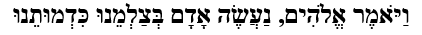It is also the value (1557) of the entire phrase from Genesis 1:26, “And God said: ‘Let us make man in our image, after our likeness;” one of the 9 Utterances. The gematria milui of this verse is 4247 or (42481), as in the 22 Names of the 22 Letters of the Alef-bet, 4248.

Oddly enough, the final letters of this phrase equal 297, the 17th Partition Number, while 22 is the 8th Partition Number.Now, 9 x 107 = 963, which is the value of the word Echad (אחד), One, spelled out. It is also the sum of the 10 first Triplets of Pi (π) whose 10 initials equal 37, as in (963 + 37) = 1000, 103, or Alef (אלף), One.  Their 10 final digits equal 63, as in (37 + 63) = 100 or 102, making those 10 Triplets connect to One, Tesla’s frequency number (963) and the structure of the Essential Cube of Creation who has the 9-6-3 pattern on two of its faces.

Equally significant is the equation of time, (963 x 12) = 11,556 years, which equals the (2 x 5778) years from the Spherical Time equation: 2(5778π) = 36304.24470, thus indicating that the time-limit radius in hyperspace is equivalent to Echad (אחד), One (1) x 12.

Moreover, while 42 x 27.5 = 1155, as in 11556, the sum of the 42 square roots of the Tetrahedron values of the 42-Letter Name is 1155.777 and the sum of the 42 square roots of the atbash values of the 42-Letter Name is 1155.57 or 1155.6.

They are all connected as One, which is why the sum of the 42 square roots of the Essential Cube order of the 42-Letter Name is 410.00963, reflecting the word Kodesh, Holy, and the first Triplet of the Shema (שמע), both 410.

### Chaos Theory

All this is happening strictly in the cosmos of hyperspace, but back in what we call physicality and set in stone blocks of granite and limestone we see the value of the 3 iterations of Ehyeh (אהיה), along with figures of otherworldly precision and another set of incredible equations.  Using the same complete 2 Pi (π) Phi(φ) cipher that gave us 5778 for the value of the Alef-bet, we get a value of 4669 for those 3 iterations of Ehyeh (אהיה). This 4669 result is highly reminiscent of Feigenbaum’s chaotic convergence coefficient, 4.669 found in an equation between the encasing pyramid’s west base and Phi(φ).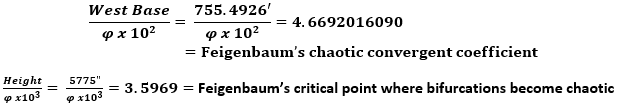The revealed codes in the encasing pyramid are only possible because it has a geometric shape. It has edges, sides, and height that can be measured. So too are the paths between the hypercubes and other cosmic networks.  As we learn to read the language of the numbers, we better understand the relationships and the importance of the paths.  Only then can we follow them.  Our brains work by building and strengthening neural pathways. Our minds work by following those pathways. Our consciousness by building and strengthening cosmic pathways. When they branch out and reach a critical mass, a tree is formed. In Genesis 3:24, the Creator placed the revolving flaming sword not at the Gate of Eden, but at the entrance to the Path of the Tree of Life.  The sword is guarding the Path until we are ready to reunite with our consciousness.